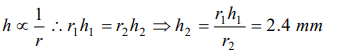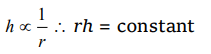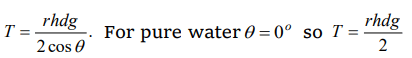## Mechanical Properties of Fluids Questions and Answers Part-16

1. Water rises upto a height h in a capillary on the surface of earth in stationary condition. Value of h increases if this tube is taken
a) On sun
b) On poles
c) In a lift going upward with acceleration
d) In a lift going downward with acceleration

Explanation:2. During capillary rise of a liquid in a capillary tube, the surface of contact that remains constant is of
a) Glass and liquid
b) Air and glass
c) Air and liquid
d) All of these

Explanation: Air and liquid

3. A shell having a hole of radius r is dipped in water. It holds the water upto a depth of h then the value of r is
a) $r=\frac{2T}{hdg}$
b) $r=\frac{T}{hdg}$
c) $r=\frac{Tg}{hd}$
d) None of these

Explanation:4. In a capillary tube, water rises by 1.2 mm. The height of water that will rise in another capillary tube having half the radius of the first, is
a) 1.2 mm
b) 2.4 mm
c) 0.6 mm
d) 0.4 mm

Explanation:5. If capillary experiment is performed in vacuum then for a liquid there
a) It will rise
b) Will remain same
c) It will fall
d) Rise to the top

Explanation: It will rise

6. If liquid level falls in a capillary then radius of capillary will
a) Increase
b) Decrease
c) Unchanged
d) None of these

Explanation:7. Water rises to a height h in a capillary at the surface of earth. On the surface of the moon the height of water column in the same capillary will be
a) 6h
b)$\frac{1}{6}h$
c) h
d) Zero

Explanation:8. Two capillary tubes of same diameter are put vertically one each in two liquids whose relative densities are 0.8 and 0.6 and surface tensions are 60 and 50 dyne/cm respectively Ratio of heights of liquids in the two tubes $\frac{h_{1}}{h_{2}}$ is
a) $\frac{10}{9}$
b) $\frac{3}{10}$
c) $\frac{10}{3}$
d) $\frac{9}{10}$

Explanation:9. Water rises in a vertical capillary tube upto a height of 2.0 cm . If the tube is inclined at an angle of $60^{\circ}$ with the vertical, then upto what length the water will rise in the tube
a) 2.0 cm
b) 4.0 cm
c) $\frac{4}{\sqrt{3}}cm$
d) $2\sqrt{2}cm$a) $\frac{\rho g}{2hr }$
b) $\frac{2}{hr\rho g }$
c) $\frac{r\rho g}{2h }$
d) $\frac{hr\rho g}{2 }$